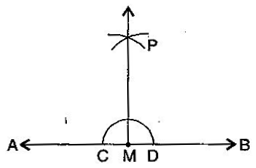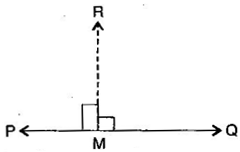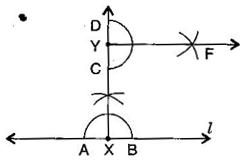### Practical Geometry-Solutions 14.4

CBSE Class –VI Mathematics
NCERT Solutions
Chaper 14 Practical Geometry (Ex. 14.4)

Question 1. Draw any line segment $\overline{\text{AB}}.$ Mark any point M on it. Through M, draw a perpendicular to $\overline{\text{AB}}.$ (Use ruler and compasses)
(i) With M as centre and a convenient radius, draw an arc intersecting the line AB at two points C and D.
(ii) With C and D as centres and a radius greater than MC, draw two arcs, which cut each other at P.
(iii) Join PM. Then PM is perpendicular to AB through the point M.Question 2. Draw any line segment $\overline{\text{PQ}}.$ Take any point R not on it. Through R, draw a perpendicular to $\overline{\text{PQ}}.$ (Use ruler and set-square)
(i) Place a set-square on $\overline{\text{PQ}}$ such that one arm of its right angle aligns along $\overline{\text{PQ}}$.
(ii) Place a ruler along the edge opposite to the right angle of the set-square.
(iii) Hold the ruler fixed. Slide the set square along the ruler till the point R touches the other arm of the set square.
(iv) Join RM along the edge through R meeting $\overline{\text{PQ}}$ at M. Then RM $\mathrm{\perp }$ PQ.Question 3. Draw a line $l$ and a point X on it. Through X, draw a line segment $\overline{\text{XY}}$ perpendicular to $l.$
Now draw a perpendicular to $\overline{\text{XY}}$ to Y. (use ruler and compasses)
(i) Draw a line ${}^{\prime }{l}^{\prime }$ and take point X on it.
(ii) With X as centre and a convenient radius, draw an arc intersecting the line ${}^{\prime }{l}^{\prime }$ at two points A and B.
(iv) Join XC and produce it to Y. Then XY is perpendicular to ${}^{\prime }{l}^{\prime }$.